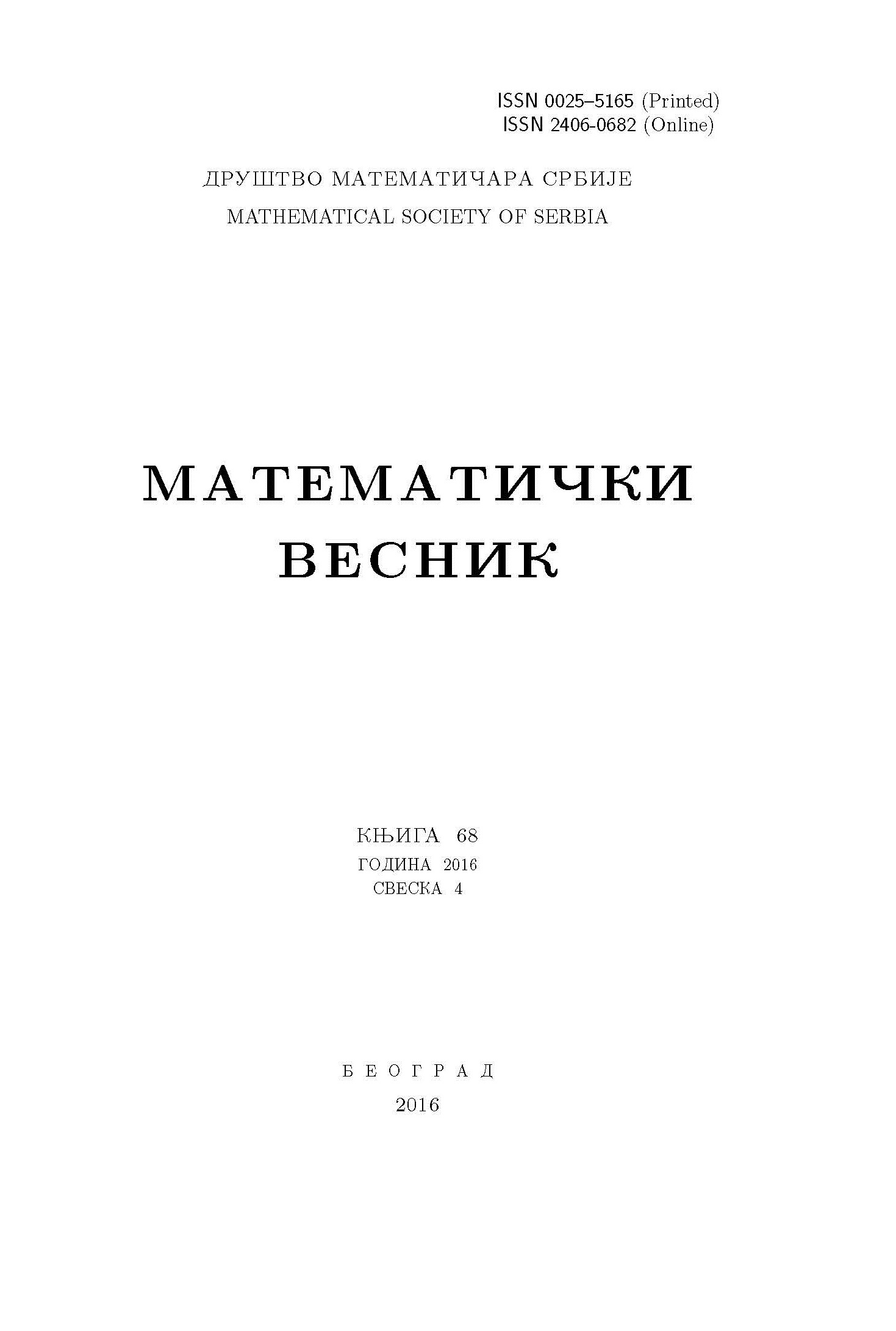﻿ Matematički Vesnik ﻿
 MATEMATIČKI VESNIK МАТЕМАТИЧКИ ВЕСНИКTHE BOREL MAPPING OVER SOME QUASIANALYTIC LOCAL RINGS M. Berraho AbstractLet $M={(M_{j})}_{j}$ be an increasing sequence of positive real numbers with $M_{0}=1$ such that the sequence $M_{j+1}/M_j$ increases and let $\mathcal{{E}}_{n}(M)$ be the Denjoy-Carleman class associated to this sequence. Let $\hat{\mathcal{{E}}}_{n}(M)$ denote the Taylor expansion at the origin of all elements that belong to the ring $\mathcal{{E}}_{n}(M)$. We say that $\hat{\mathcal{{E}}}_{n}(M)$ satisfies the splitting property if for each $f\in\hat{\mathcal{{E}}}_{n}(M)$ and $A \cup B =\mathbb{N}^{n}$ a partition of $\mathbb{N}^{n}$, when $G=\sum_{w\in A}a_{w}x^{w}$ and $H=\sum_{w\in B}a_{w}x^{w}$ are formal power series with $f=G+H$, then $G\in\hat{\mathcal{{E}}}_{n}(M)$ and $H\in\hat{\mathcal{{E}}}_{n}(M)$. Our first goal is to show that if the Borel mapping $^\wedge:\mathcal{{E}}_{1}(M)\rightarrow\mathbb{R}[[x_1]]$ is a homeomorphism onto its range for the inductive topologies, then the ring $\mathcal{{E}}_{1}(M)$ coincides with the ring of real analytic germs. Secondly, we will give a negative answer to the splitting property for the quasianalytic local rings $\mathcal{{E}}_{n}(M)$. In the last section, we will show that the ring of smooth germs that are definable in the polynomially bounded o-minimal structure of the real field expanded by all restricted functions in some Denjoy-Carleman rings does not satisfy the splitting property in general.Keywords: Denjoy-Carleman rings; splitting property; Borel mapping; quasianalyticity. MSC: 26E10, 03C64 Pages:  35$-$41 Volume  74 ,  Issue  1 ,  2022

﻿